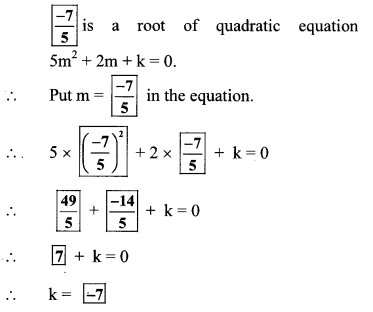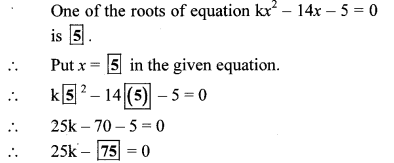# Maharashtra Board 10th Class Maths Part 1 Practice Set 2.1 Solutions Chapter 2 Quadratic Equations

Balbharti Maharashtra State Board Class 10 Maths Solutions covers the Practice Set 2.1 Algebra 10th Class Maths Part 1 Answers Solutions Chapter 2 Quadratic Equations.

## Practice Set 2.1 Algebra 10th Std Maths Part 1 Answers Chapter 2 Quadratic Equations

Question 1.
Solution:
i. y2 – 7y + 12 = 0
ii. x2 – 8 = 0

Question 2.
Decide which of the following are quadratic
i. x2 – 7y + 2 = 0
ii. y2 = 5y – 10
iii. y2 + $$\frac { 1 }{ y }$$ = 2
iv. x + $$\frac { 1 }{ x }$$ = -2
v. (m + 2) (m – 5) = 03
vi. m3 + 3m2 – 2 = 3m3
Solution:
i. The given equation is x2 + 5x – 2 = 0
Here, x is the only variable and maximum index of the variable is 2.
a = 1, b = 5, c = -2 are real numbers and a ≠ 0.
∴ The given equation is a quadratic equation.

ii. The given equation is
y2 = 5y – 10
∴ y2 – 5y + 10 = 0
Here, y is the only variable and maximum index of the variable is 2.
a = 1, b = -5, c = 10 are real numbers and a ≠ 0.
∴ The given equation is a quadratic equation.

iii. The given equation is
y2 + $$\frac { 1 }{ y }$$ = 2
∴ y3 + 1 = 2y …[Multiplying both sides by y]
∴ y3 – 2y + 1 = 0
Here, y is the only variable and maximum index of the variable is not 2.
∴ The given equation is not a quadratic equation.

iv. The given equation is
x + $$\frac { 1 }{ x }$$ = -2
∴ x2 + 1 = -2x …[Multiplying both sides by x]
∴ x2 + 2x+ 1 = 0
Here, x is the only variable and maximum index of the variable is 2.
a = 1, b = 2, c = 1 are real numbers and a ≠ 0.
∴ The given equation is a quadratic equation.

v. The given equation is
(m + 2) (m – 5) = 0
∴ m(m – 5) + 2(m – 5) = 0
∴ m2 – 5m + 2m – 10 = 0
∴ m2 – 3m – 10 = 0
Here, m is the only variable and maximum index of the variable is 2.
a = 1, b = -3, c = -10 are real numbers and a ≠ 0.
∴ The given equation is a quadratic equation.

vi. The given equation is
m3 + 3m2 – 2 = 3m3
∴ 3m3 – m3 – 3m2 + 2 = 0
∴ 2m3 – 3m2 + 2 = 0
Here, m is the only variable and maximum
index of the variable is not 2.
∴ The given equation is not a quadratic equation.

Question 3.
Write the following equations in the form ax2 + bx + c = 0, then write the values of a, b, c for each equation.
i. 2y = 10 – y2
ii. (x – 1)2 = 2x + 3
iii. x2 + 5x = – (3 – x)
iv. 3m2 = 2m2 – 9
v. P (3 + 6p) = – 5
vi. x2 – 9 = 13
Solution:
i. 2y – 10 – y2
∴ y2 + 2y – 10 = 0
Comparing the above equation with
ay2 + by + c = 0, we get
a = 1, b = 2, c = -10

ii. (x – 1)2 = 2x + 3
∴ x2 – 2x + 12x + 3
x2 – 2x + 1 – 2x – 30
∴ x2 – 4x – 2 = 0
Comparing the above equation with
ax2 + bx + c = 0, we get
a = 1, b = -4, c = -2

iii. x2 + 5x = – (3 – x)
∴ x2 + 5x = -3 + x
∴ x2 + 5x – x + 3 = 0
∴ x2 + 4x + 3 = 0
Comparing the above equation with
ax2 + bx + c = 0, we get
a = 1, b = 4, c = 3

iv. 3m2 = 2m2 – 9
∴ 3m2 – 2m2 + 9 = 0
∴ m2 + 9 = 0
∴ m2 + 0m + 9 = 0
Comparing the above equation with
am2 + bm + c = 0, we get
a = 1, b = 0, c = 9

v. p (3 + 6p) = – 5
∴ 3p + 6p2 = -5
∴ 6p2 + 3p + 5 = 0
Comparing the above equation with
ap2 + bp + c = 0, we get
a = 6, b = 3, c = 5

vi. x2 – 9 = 13
∴ x2 – 9 – 13 = 0
∴ x2 – 22 = 0
∴ x2 + 0x – 22 = 0
Comparing the above equation with
ax2 + bx + c = 0, we get
a = 1, b = 0, c = -22

Question 4.
Determine whether the values given against each of the quadratic equation are the roots of the equation.
i. x2 + 4x – 5 = 0; x = 1,-1
ii. 2m2 – 5m = 0; m = 2, $$\frac { 5 }{ 2 }$$
Solution:
i. The given equation is
x2 + 4x – 5 = 0 …(i)
Putting x = 1 in L.H.S. of equation (i), we get
L.H.S. = (1)2 + 4(1) – 5 = 1 + 4 – 5 = 0
∴ L.H.S. = R.H.S.
∴ x = 1 is the root of the given quadratic equation.
Putting x = -1 in L.H.S. of equation (i), we get
L.H.S. = (-1)2 + 4(-1) – 5 = 1 – 4 – 5 = -8
∴ LH.S. ≠ R.H.S.
∴ x = -1 ¡s not the root of the given quadratic equation.

ii. The given equation is
2m2 – 5m = 0 …(i)
Putting m = 2 in L.H.S. of equation (i), we get
L.H.S. = 2(2)2 – 5(2) = 2(4) -10 = 8 – 10 = -2
∴ L.H.S. ≠ R.H.S.
∴ m = 2 is not the root of the given quadratic equation.
Putting m = $$\frac { 5 }{ 2 }$$ in L.H.S. of equation (i), we getQuestion 5.
Find k if x = 3 is a root of equation kx2 – 10x + 3 = 0.
Solution:
x = 3 is the root of the equation kx2 – 10x + 3 = 0.
Putting x = 3 in the given equation, we get
k(3)2 – 10(3) + 3 = 0
∴ 9k – 30 +3 = 0
∴ 9k – 27 = 0
∴ 9k = 27
∴ k = $$\frac { 27 }{ 9 }$$
∴ k = 3

Question 6.
One of the roots of equation 5m2 + 2m + k = 0 is $$\frac { -7 }{ 5 }$$ Complete the following activity to find the value of ‘k’.
Solution:Question 1.
x2 + 3x – 5, 3x2 – 5x, 5x2; Write the polynomials In the index form. Observe the coefficients and fill in the boxes. (Textbook p. no. 31)
Index form of the given polynomials:
x2 + 3x – 5, 3x2 – 5x + 0, 5x2 + 0x + 0
i. Coefficients of x2 are ,  and  respectively, and these coefficients are non zero.
ii. Coefficients of x are 3, [-5] and  respectively.
iii. Constant terms are [-5],  and  respectively.
Here, constant terms of second and third polynomial is zero.

Question 2.
Complete the following table (Textbook p. no. 31)Question 3.
Decide which of the following are quadratic equations? (Textbook pg. no. 31)
i. 9y2 + 5 = 0
ii. m3 – 5m2 + 4 = 0
iii. (l + 2)(l – 5) = 0
Solution:
i. In the equation 9y2 + 5 = 0, [y] is the only variable and maximum index of the variable is .
∴ It [is] a quadratic equation.

ii. In the equation m3 – 5m2 + 4 = 0, [m] is the only variable and maximum index of the variable is not 2.
∴ It [is not] a quadratic equation.

iii. (l + 2)(l – 5) = 0
∴ l(l – 5) + 2(l – 5) = 0
∴ l2 – 5l + 2l – 10 = 0
∴ l2 – 3l – 10 = 0.
In this equation [l] is the only variable and maximum index of the variable is 
∴ it [is] a quadratic equation.

Question 4.
If x = 5 is a root of equation kx2 – 14x – 5 = 0, then find the value of k by completing the following activity. (Textbook pg, no. 33)
Solution: